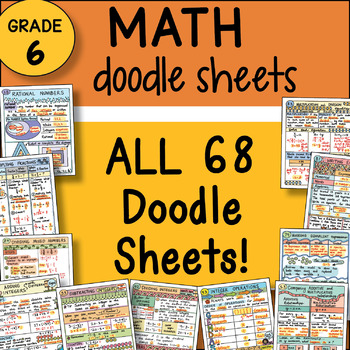DID YOU KNOW:
Seamlessly assign resources as digital activities

Learn how in 5 minutes with a tutorial resource. Try it Now

Learn More# 6th Grade Math Interactive Notebook DOODLE SHEETS ~ Fun and Engaging Notes5th - 7th, Homeschool
Subjects
Resource Type
Formats Included
• Zip
Pages
1200 +
\$49.00
List Price:
\$204.00
You Save:
\$155.00
\$49.00
List Price:
\$204.00
You Save:
\$155.00

### Description

To sample this product: try this FREEBIE: Doodle Sheet - Prime Factorization

68 Math Doodle Sheets - explains each math concept

68 Guided Practice Sheets to check for understanding of each concept

68 PowerPoints to show the key to the doodle notes and the guided practice!

* Two versions of each sheet included - Full sheet and INB Size

* TEKS AND CC Aligned! Full Year Alignment page included.

These 6th grade math doodle sheets are a great way to help the students learn math concepts! Students are engaged as they take creative notes and decorate to make them their own. Doodling helps content retention and students love these! Students will have the most complete creative math interactive notebook ever! As an added bonus, so many times parents want to help but don’t understand how to do math anymore and these descriptive step by step sheets help students and parents understand math. This interactive notebook works as an amazing resource to refer to for the whole year!

LIST of 68 Doodle Sheets plus Guided Practice Sheets

1. The Number System

2. Integers and Their Opposites

3. Comparing and Ordering Integers

4. Absolute Value

5. Rational Numbers

6. Opposites and Absolute Value of Rational Numbers

7. Comparing and Ordering Rational Numbers

8. Multiplying Fractions

9. Multiplying Mixed Numbers

10. Dividing Fractions

11. Dividing Mixed Numbers

12. Multiplying Decimals with Models

13. Multiplying Decimals with the Standard Algorithm

14. Dividing Decimals with Models

15. Dividing Decimals with the Standard Algorithm

16. Multiplying and Dividing Fractions and Decimals

17. Checking with the Digital Root

18. Adding Integers with the Same Sign

19. Adding Integers with Different Signs

20. Subtracting Integers

22. Multiplying Integers

23. Dividing Integers

24. Integer Operations

25. Ratios

26. Rates

27. Comparing Additive and Multiplicative Relationships

28. Ratios and Rates in Tables and Graphs

29. Converting Measurements

30. Understanding Percent

31. Benchmark Fractions to Decimals

32. Percents with Fractions and Decimals

33. Percent Problems

34. Exponents

35. Prime Factorization

36. Order of Operations

37. Modeling Equivalent Expressions

38. Evaluating Equivalent Expressions

39. Generating Equivalent Expressions

40. Writing Equations

42. Multiplication and Division Equations

43. Writing Inequalities

45. Multiplication & Division of Inequalities with Positive Numbers

46. Multiplication & Division of Inequalities with Rational Numbers

47. Graphing and the Coordinate Plane

48. Independent and Dependent Variables

49. Writing Equations from Tables

50. Algebraic Relationships in Tables and Graphs

51. Forming Triangles

52. Sum of Angle Measures

53. Relationships of Sides and Angles

55. Area of Triangles

56. Solving Area Equations

57. Solving Volume Equations

58. Measures of Center (Mean, Median, and Range)

59. Box Plots

60. Dot Plots

61. Stem and Leaf Plots & Histograms

62. Categorizing Data

63. Checking Accounts

64. Balancing a Check Register

65. Credit and Debit Cards

66. Credit Reports

67. Paying for College

68. Wages, Salaries and Careers

Total Pages
1200 +
Included
Teaching Duration
1 Year
Report this Resource to TpT
Reported resources will be reviewed by our team. Report this resource to let us know if this resource violates TpT’s content guidelines.